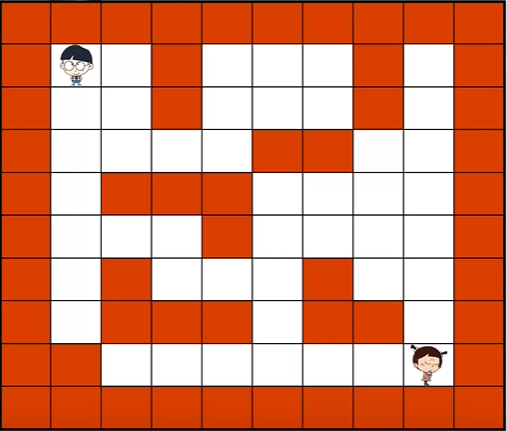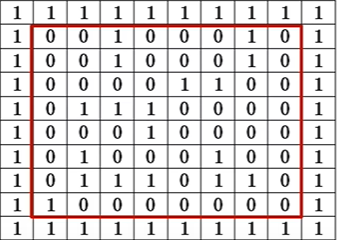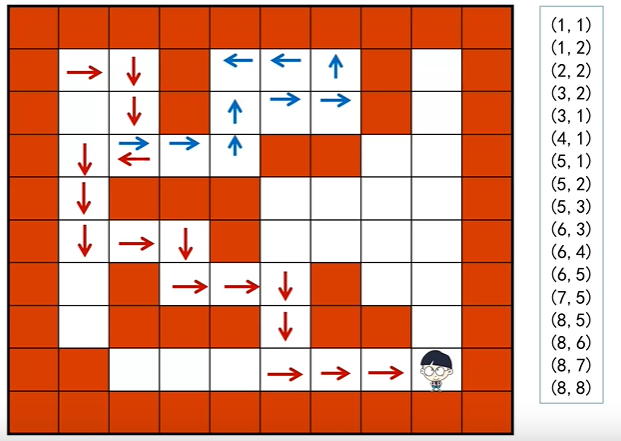# 迷宫求解(DFS)，栈实现，数据结构笔记

## 迷宫求解深度优先(DFS)，栈实现maze[x][y] = 0:该位置没走过且不是障碍
maze[x][y] = 1:障碍
maze[x][y] = -1:该位置走过了

方向试探：
typedef struct{
int incX,incY
}Direction
Direction direct;

line=x+direct[v].incX;
col=y+direct[v].incY;
direct:incX=0,incY=1     向右走一步
direct:incX=1,incY=0     向下走一步
direct:incX=0,incY=-1    向左走一步
direct:incX=-1,incY=0    向下走一步

typedef struct{
int x,y         当前访问的迷宫格子的坐标；
int di;         当前方向
}Box;           //用于记录当前坐标以及下一步所走的方向


bool findPath(int maze[M+2][N+2], Direction direct[],Stack &s)
{
Box temp;
int x,y,di;
int line col;
maze=-1;
temp={1,1,-1};
s.push(s,temp)
while(!s.isEmpty())
{
temp=s.pop;                                        //退一步
x=temp.x;y=temp.y;di=temp.di+1;
while(di<4)
{
line=x+direct[di].incX;
col=x+direct[di].incY;
if(maze[line][col]==0)             				//下一步没走过且不是障碍
{
temp={x,y,di}
s.push(temp);			  				 //把这一步的位置和方向全部压栈
x=line; y=col maze[line][col]=-1		//移动到下一步
if(x==M&&y=N) return true;				//如果移动到终点，结束程序
else di=0;                              //方向重置
}
else di++;										//换一个方向
}
}

return false;
}06-023515
03-19516903-26271
04-09186
09-24682
03-17109
01-181340
03-30145
01-17713
03-04171